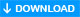# fgetcsv(): Getting data from CSV file

``fgetcsv(f_pointer,int \$length,string \$delimiter, string \$encloser,string \$escape);``
ParameterDESCRIPTION
f_pointerRequired : a successful file pointer
\$lengthOptional : Must be greater than the maximum line length
\$delimiterOptional : One char only
\$encloserOptional : Field encloser char
\$escapeOptional : escape parmeter sets the escape char
We can get data from a CSV file (comma-separated values ) by using fgetcsv() function in PHP. This string function returns us line of data or a record as an array. This function reads the line from the file pointer we provide ( by fopen ) and parse for CSV data. So data can be collected as elements of the array.

Output is indexed array containing the fields read.

## Example of fgetcsv()

We will use our student table csv data. ( Download a copy of student.csv file at end of this tutorial )
You can read how the CSV data is prepared from the student table here.
Now the student data is available to us in a csv file

We will first open the csv file and keep the pointer
``\$f_pointer=fopen("student.csv","r"); // file pointer``
Then we will use the pointer to loop through all the rows of the file till the end of the file is reached. Inside the loop we will use fgetcsv() to collect the array and then print the output. Here is the code.
``````<?php
\$f_pointer=fopen("student.csv","r"); // file pointer

while(! feof(\$f_pointer)){
\$ar=fgetcsv(\$f_pointer);
echo print_r(\$ar); // print the array
echo "<br>";
}
?>``````
The output is here.
``````Array (  => 1  => John Deo  => Four  => 75  => female )
Array (  => 2  => Max Ruin  => Three  => 85  => male )
Array (  => 3  => Arnold  => Three  => 55  => male )
Array (  => 4  => Krish Star  => Four  => 60  => female )
Array (  => 5  => John Mike  => Four  => 60  => female )
Array (  => 6  => Alex John  => Four  => 55  => male )
``````

## Generating a SQL file to insert records into database

Now we can separate data from the above code and using the data we will prepare series of SQL insert commands to store or add all these records to student table. Each loop will have one insert command. Here is the code.
``````<?php
\$f_pointer=fopen("student.csv","r"); // file pointer

while(! feof(\$f_pointer)){
\$ar=fgetcsv(\$f_pointer);
\$sql="INSERT INTO student(id,name,class,mark,sex)values('\$ar','\$ar','\$ar','\$ar','\$ar')";
echo \$sql;
echo "<br>";
}
?>
``````
Part of the output is here
``````INSERT INTO student(id,name,class,mark,sex)values('1','John Deo','Four','75','female')
INSERT INTO student(id,name,class,mark,sex)values('2','Max Ruin','Three','85','male')
INSERT INTO student(id,name,class,mark,sex)values('3','Arnold','Three','55','male')
INSERT INTO student(id,name,class,mark,sex)values('4','Krish Star','Four','60','female')``````

## Removing First line as column headers

In a csv file we may like not to use first line as data as they will have column headers.
``````<?php
\$f_pointer=fopen("student.csv","r"); // file pointer
\$first_line="T";

while(! feof(\$f_pointer)){
\$ar=fgetcsv(\$f_pointer);
if(\$first_line<>'T'){
\$sql="INSERT INTO student(id,name,class,mark,sex)values('\$ar','\$ar','\$ar','\$ar','\$ar')";
echo \$sql;
echo "<br>";
}
\$first_line='F';
}
?>``````
If you want the sql file to be downloaded then follow the instruction at the end of CSV file creation.

fputcsv(): to write data to a csv filestudent.csvSubscribe to our YouTube Channel here

## Subscribe

* indicates required
Subscribe to plus2netplus2net.com

PHP String Functions

Post your comments , suggestion , error , requirements etc here .